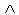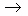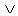Logic
edHelper subscribers - Create a new printable

Number of Keys
 Select the number of different printables: 1 key 2 keys 3 keys 4 keys 5 keys

Number of Pages (for each key)
 Select the number of pages: 1 page 2 pages 3 pages 4 pages

Include an answer key (answer keys will be at the end of the printable)
High School Geometry
High School Geometry
Math

 Name _____________________________Date ___________________
Logic
State the truth value of the following statements.

 1 If p is true and q is true, state the truth for the converse of:(~ p~ q )( pq )
 2 If p is true and q is false, state the truth for the contrapositive of:( p~ q )( qp )
 3 If p is true and q is false, state the truth for the inverse of: p(~ q~ p )
 4 If p is false and q is true, state the truth for the converse of:( q~ p )( pq )
 5 If p is true and q is true, state the truth for the inverse of:( pq )q
 6 If p is true and q is true, state the truth for the contrapositive of:( p~ q )q
 7 If p is false and q is false, state the truth for the contrapositive of:(~ q~ p )(~ q~ p )
 8 If p is true and q is true, state the truth for the inverse of: p(~ q~ p )
 9 If p is true and q is true, state the truth for the converse of:(~ pq )(~ q~ p )
 10 If p is false and q is true, state the truth for the converse of:~(( pq )q )
 11 If p is true and q is true, state the truth for the inverse of:(~ pq )( qp )
 12 If p is true and q is true, state the truth for the contrapositive of: p( pq )
 13 If p is false and q is true, state the truth for the contrapositive of:( p~ q )(~ pq )
 14 If p is true and q is false, state the truth for the converse of:~(( qp )( qp ))
 15 If p is false and q is true, state the truth for the inverse of:( q~ p )q
 16 If p is false and q is true, state the truth for the contrapositive of:(~ qp )q

Sample
This is only a sample worksheet.

Logic
edHelper subscribers - Create a new printable

Number of Keys
 Select the number of different printables: 1 key 2 keys 3 keys 4 keys 5 keys

Number of Pages (for each key)
 Select the number of pages: 1 page 2 pages 3 pages 4 pages